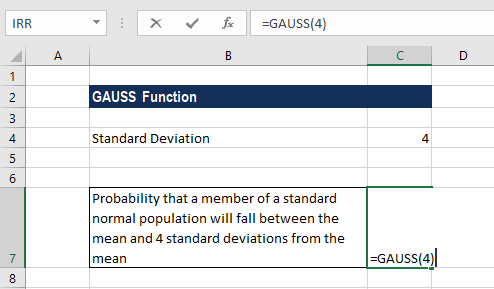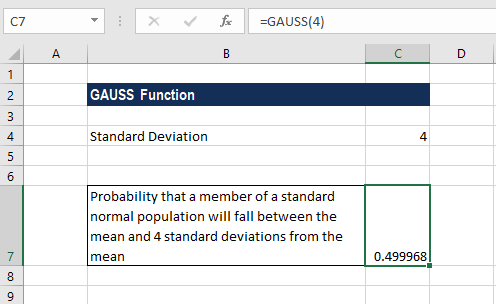# GAUSS Function

Probability that a member of a standard normal population will be between the mean and a specified number of standard deviations

## What is the GAUSS Function?

The GAUSS function is categorized under Excel Statistical functions. It will return the probability that a member of a standard normal population will be between the mean and a specified number of standard deviations from the mean. In other words, GAUSS gives us a way to solve a specific case where the range goes from the mean to a point above the mean.

As a financial analyst, the GAUSS function is useful in understanding markets, prices, and probabilities. For example, if we wish to invest \$5,000 in stocks and bonds, with annual return rates of 15% and 6%, respectively, we can use the function to find out how to allocate our investment capital to get the desired return(s).

GAUSS was introduced in MS Excel 2013 and is not available in earlier versions. The function is capable of efficiently handling large datasets and providing tools for easy data management, making it suitable for conducting analysis using high frequency, real-time data.

### Gauss Formula

=GAUSS(z)

The formula uses only one argument:

1. Z (required argument) – This is the real number at which we want to evaluate the GAUSS function.

### How to use the GAUSS Function in Excel?

To understand the uses of the GAUSS function, let’s consider an example:

#### Example

Suppose we wish to calculate the probability that a member of a standard normal population will fall between the mean and four standard deviations from the mean. The formula to use for calculating the same will be:We get the result below:### Things to remember about the GAUSS Function

1. #VALUE! error – Occurs when any of the number arguments that are provided directly to the function are text strings that cannot be interpreted as numbers – that is, the value provided for Z is non-numeric.
2. #NUM! error – Occurs when the given value of Z is an invalid number.
3. As NORM.S.DIST(0,True) always returns 0.5, GAUSS(z) will always be 0.5 less than NORM.S.DIST(z,True).
4. The GAUSS function is not particularly meaningful for negative values of z. To calculate the probability that something falls in the range of -1.5 to the mean, we need to use the formula =GAUSS(1.5).
5. If we use Excel 2010 or earlier versions, the formula is =NORM.S.DIST(z,True)-0.5.

Thanks for reading CFI’s guide to important Excel functions! By taking the time to learn and master these functions, you’ll significantly speed up your financial analysis. To learn more, check out these additional CFI resources:

• Excel Functions for Finance